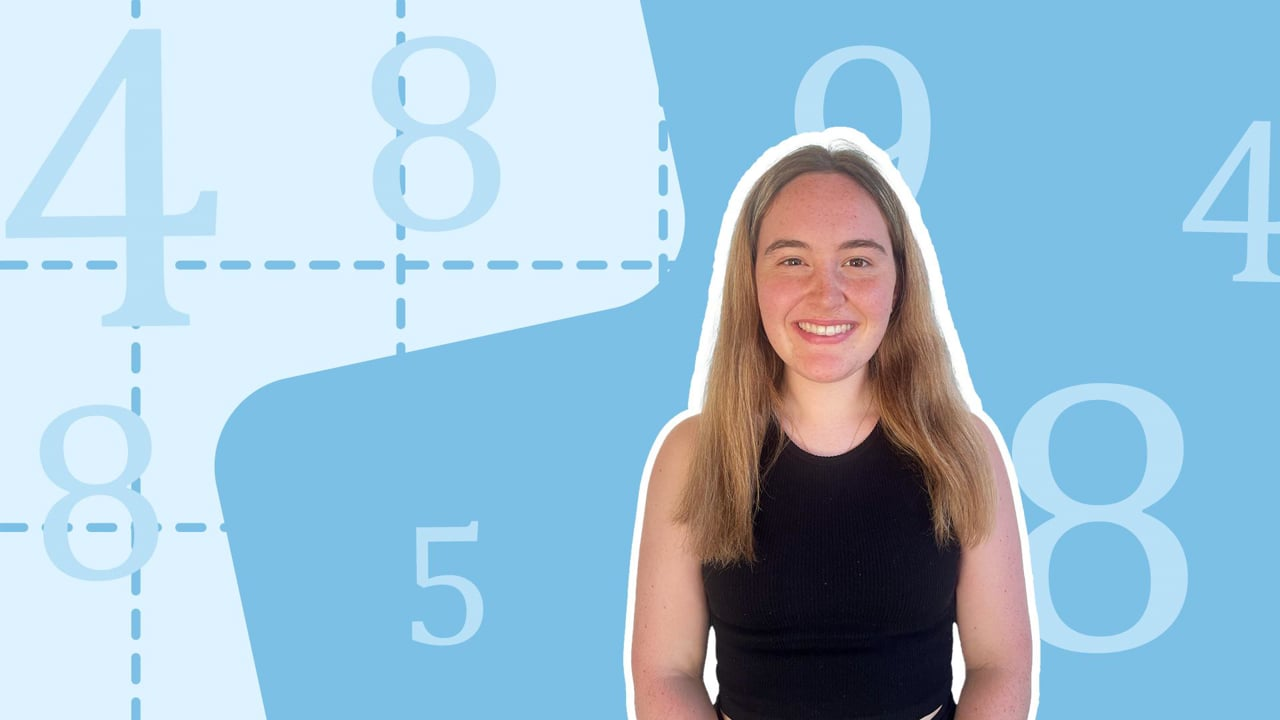Chapter OverviewMaths

Number and place value

Multiplication and division

Fractions

Measurement

Geometry - properties of shapes

Statistics

Maths

# Comparing decimals0%

Summary

# Comparing decimals

## ​​In a nutshell

Being able to compare the sizes of different decimals allows them to be placed in order. A number line can be useful to help visualise this process.

## Which is bigger?

In order to decide which number is larger, its placement and position on a number line can be looked at. The further up the number line it is, the bigger its size.

##### Example 1

Which is bigger: $4.2$ or $4.7$?

$\begin{array}{cccccccccccccc}&&&& \\& &&&\downarrow &&&&&&&&&&\downarrow &&&&\\\bold4&&4.1&&\underline{4.2}&&4.3&&4.4&&4.5&&4.6&&\underline{4.7}&&4.8 &&4.9&&\bold5\\|&&|&&|&&|&&|&&|&&|&&|&&|&&|&&| \\ \hline\end{array}$​​

Notice how $4.7$ is positioned further up the number line than $4.2$.​

What's more, $4.2$ and $4.7$ both share the same digit in their ones column whilst the digits in the tenths column differ.

$7$​ is greater than $2$ and so:

$4.7$ is greater than $4.2$.

## Ordering decimals

To order a set of decimals, compare them with each other and decide which are bigger.

#### Procedure

 1 Look at the digits in each place value column, one at a time from left to right. 2 If the digits are the same, move on to the next place value column.If the digits are different, place them (and the number associated with them) in order the required order. 3 Continue the process until all the decimals are arranged in order.

##### Example 2

Place the numbers $1.72, 2.52, 1.68$ and $1.73$ in order from smallest to largest.

Look at the digits in the ones column: $1,2,1$ and $1$.

$2$​ is the only differing digit. It is bigger than $1$so $2.52$ is the largest number.

Look at the remaining digits in the tenths column: $7,6$ and $7.$

$6$​ is the only differing digit. It is smaller than $7$​, so $1.68$ is less than $1.72$ and $1.73$.

Look at the remaining digits in the hundredths column: $2$ and $3$.

$3$​ is bigger than $2$​ so $1.73$ is greater than $1.72$.

Using the information gathered, place all four numbers in order.

$\underline{1.68,1.72,1.73,2.52}$

## Want to find out more? Check out these other lessons!

Rounding decimals

FAQs

• Question: How can I use a number line to decide which decimal is bigger?

Answer: In order to decide which number is larger, its placement and position on a number line can be looked at. The further up the number line it is, the bigger its size.

• Question: How do I order decimals?

Answer: To order a set of decimals, compare them with each other and decide which are bigger: 1. Look at the digits in each place value column, one at a time from left to right. 2. If the digits are: - the same, move on to the next place value column. - different, place them (and the number associated with them) in order the required order. 3. Continue the process until all the decimals are arranged in order.

Theory

Exercises